# GED Math : Solving for the Variable

## Example Questions

1 2 3 4 5 6 7 9 Next →

### Example Question #81 : Solving For The Variable

Solve: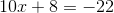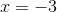Explanation:

Subtract the 8 from the left and add (subtract) from the right: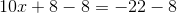Simplify: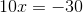Divide by 10: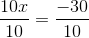Simplify:### Example Question #81 : Solving For The Variable

Solve: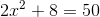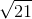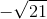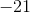Explanation:

Step 1: Move the constant from the left to the right: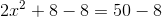Step 2: Simplify: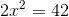Step 3: Divide by the coefficient on the left hand side to both sides of the equation: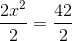Simplify: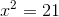Step 4: Take the square root of both sides: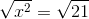Simplify: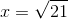1 2 3 4 5 6 7 9 Next →

### All GED Math Resources